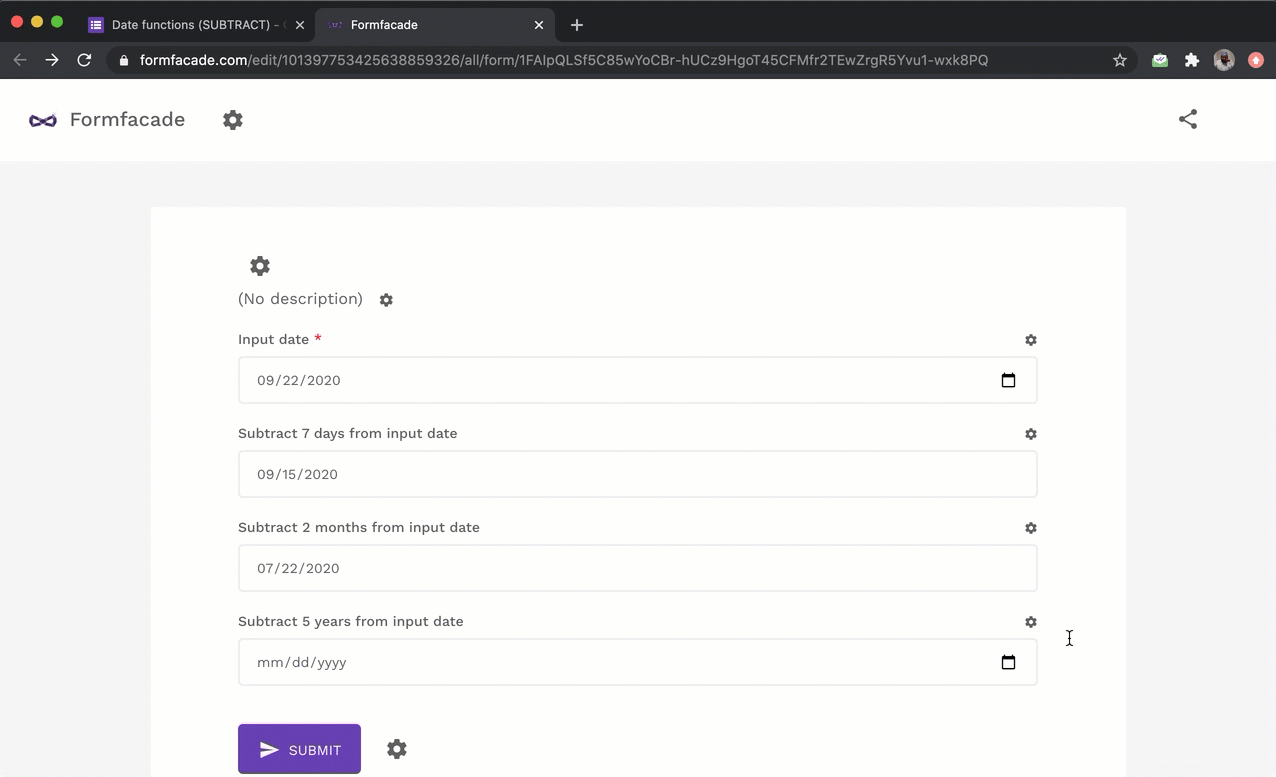### You can use the SUBTRACT function to subtract days, months or years from a date. The subtract function can be used with an input date field or a calculated date field.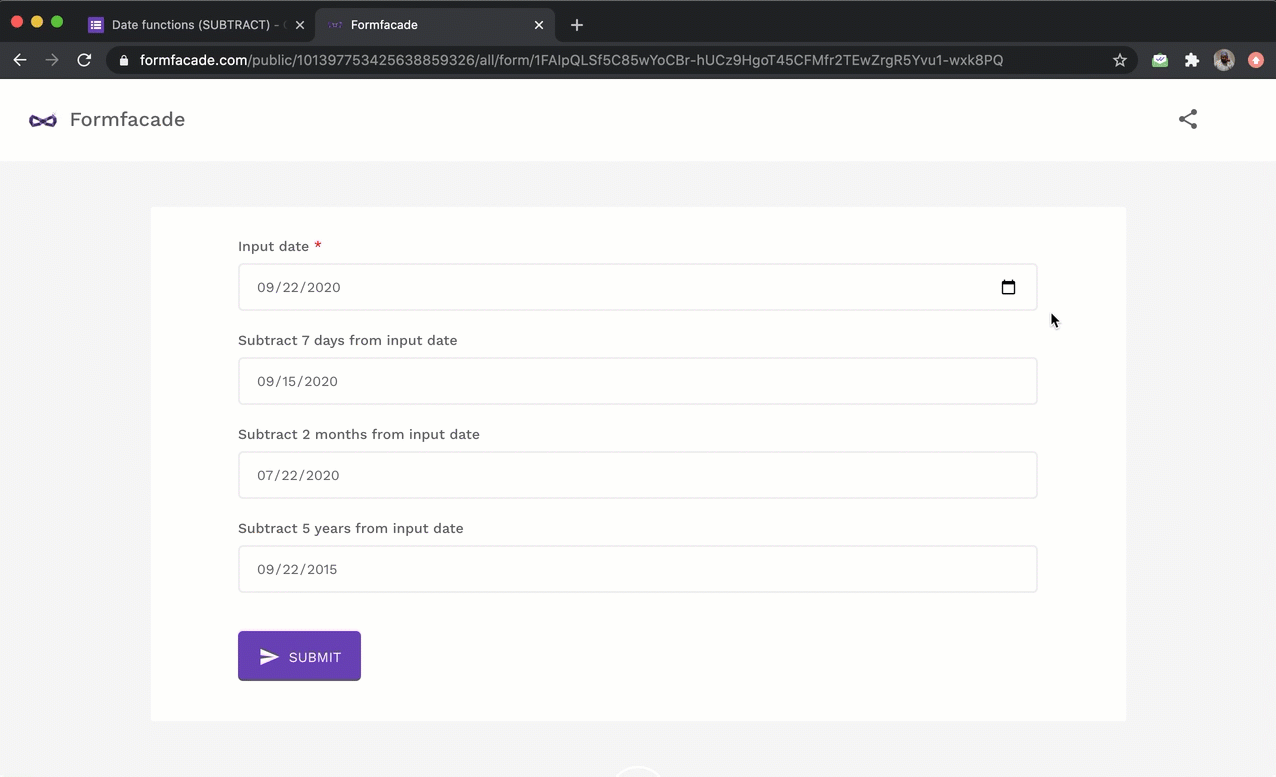#### Subtract days from a date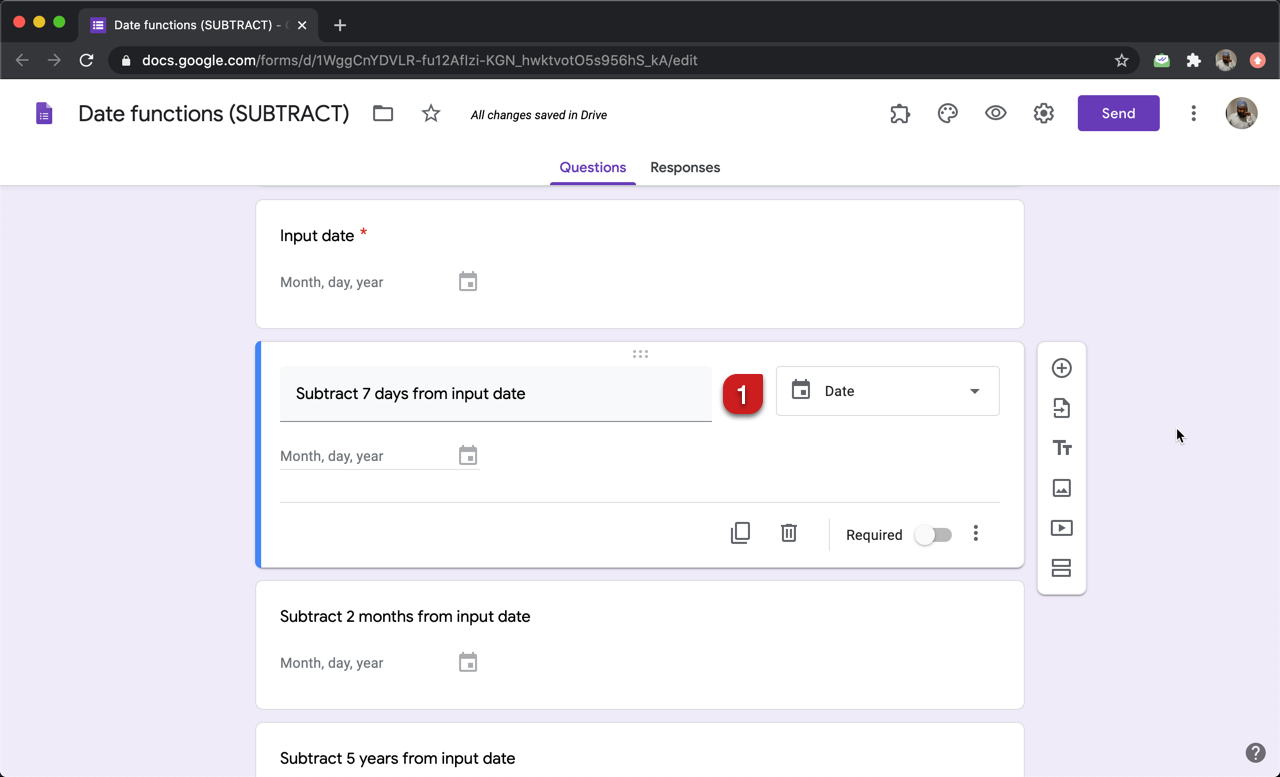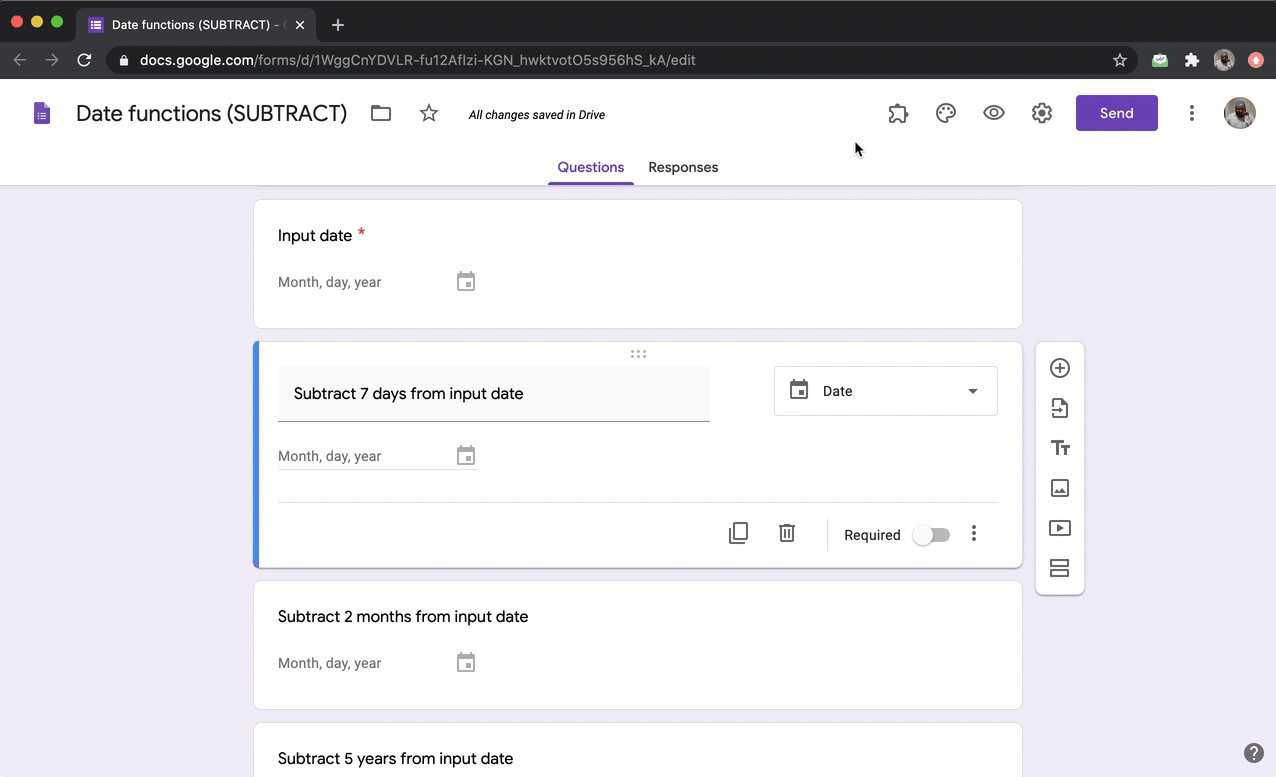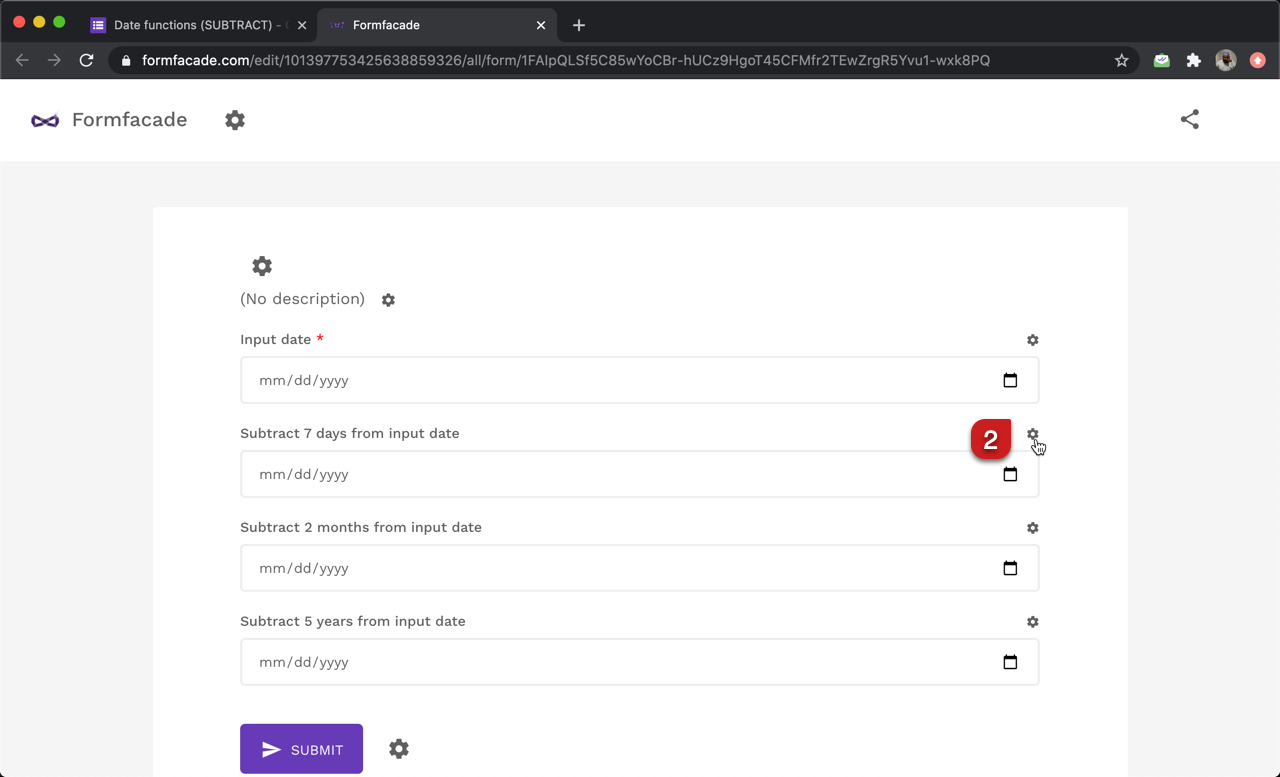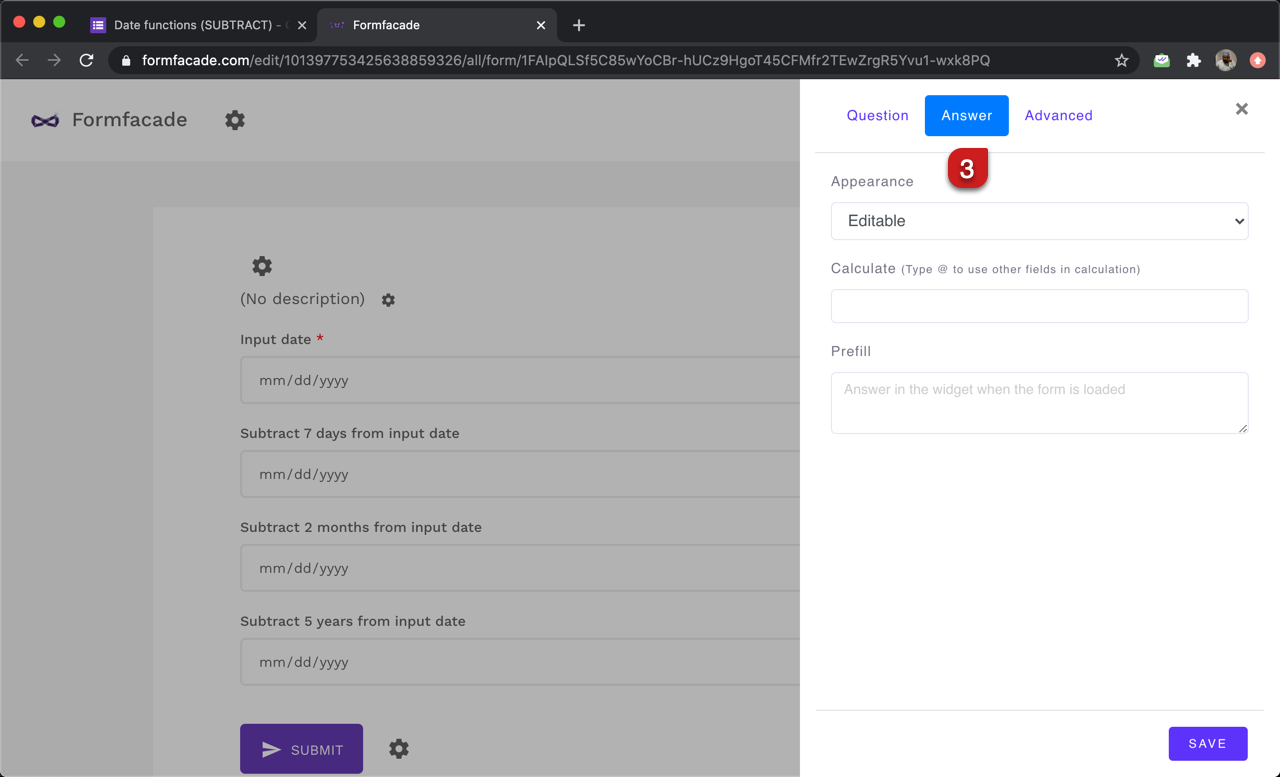#### SUBTRACT function

You can subtract days from a date by specifying "days" as the term in the SUBTRACT function.

Syntax:
subtract(number_of_days, "days")

Example usage:
TODAY().subtract(30,"days")
Inputdate.subtract(7,"days")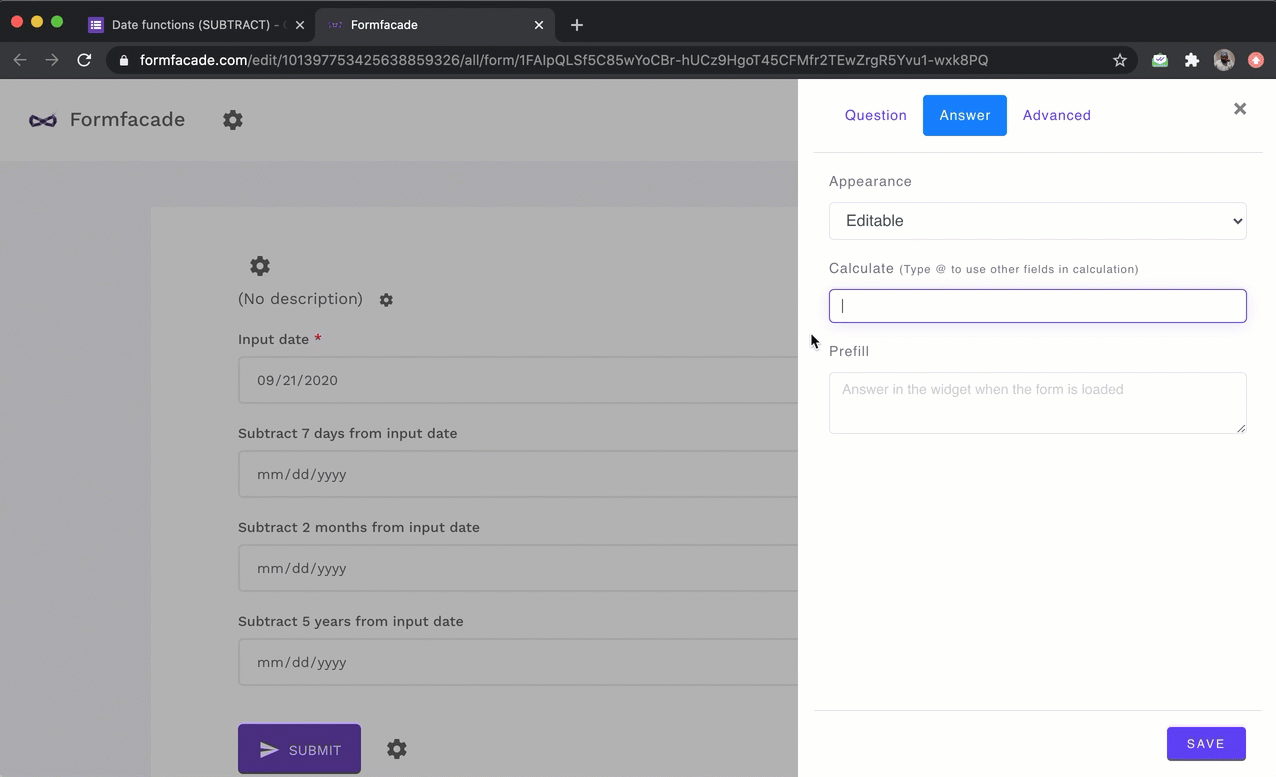#### Subtract months from a date

You can subtract months from a date by specifying "months" as the term in the SUBTRACT function.

Syntax:
subtract(number_of_months, "months")

Example usage:
TODAY().subtract(6,"months")
Inputdate.subtract(2,"months")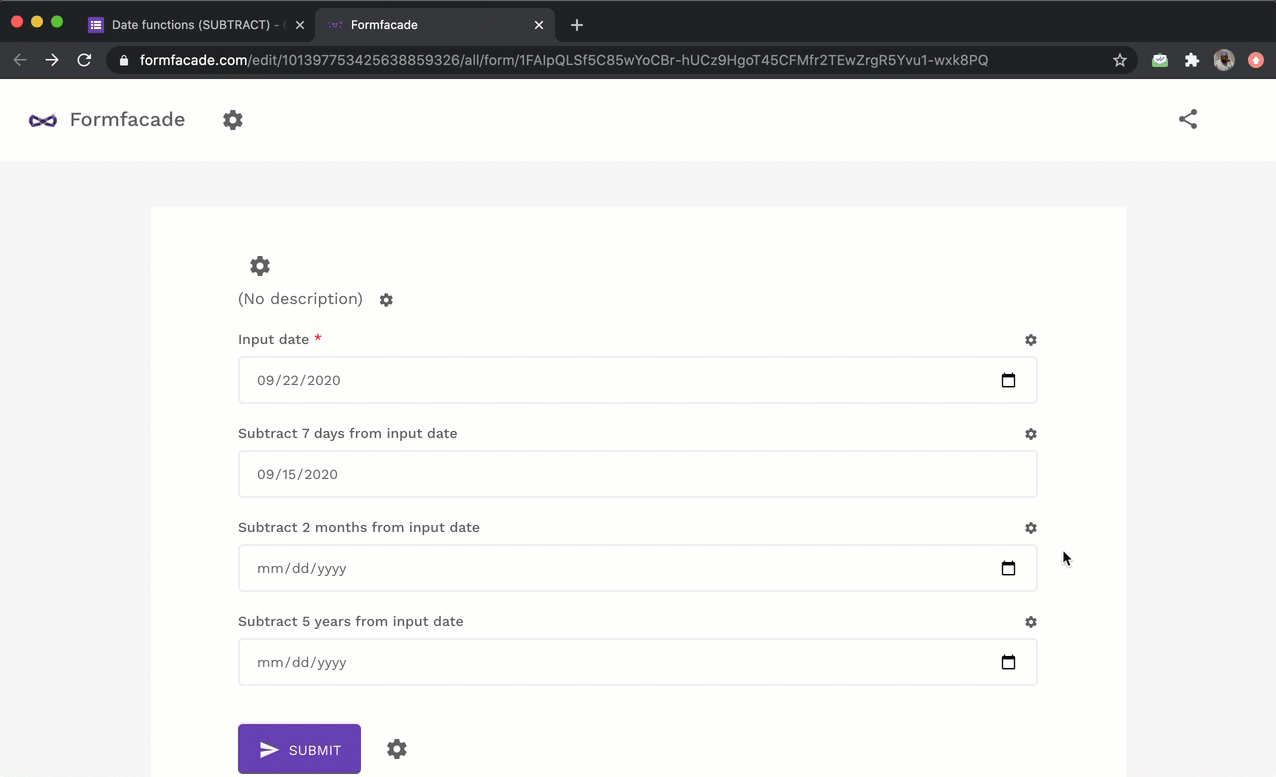#### Subtract years from a date

You can subtract years from a date by specifying "years" as the term in the SUBTRACT function.

Syntax:
subtract(number_of_years, "years")

Example usage:
TODAY().subtract(1,"years")
Inputdate.subtract(5,"years")